8.1

## GTP plot

Tools for visualizing the performance of a gradual typing system. If you have performance data, this package should help you plot it.

### 1Introduction: Gradual Typing Performance

A gradual typing system lets programs mix dynamically typed and statically typed code.
• dynamically typed code avoids type errors at run-time, using run-time checks.

• statically typed code avoids type errors at compile-time, using ahead-of-time checks.

Typed Racket is a gradual typing system because programs can mix statically-typed typed/racket code with dynamically-typed racket code.

The main challenge of gradual typing is: how to protect the assumptions of statically typed code from dynamically typed code? One way to do this is to use run-time checks:
• if statically typed code is expecting a value with type T,

• and receives a dynamically typed value v,

• then check something like (if (T? v) v (error 'bad-type))

A run-time check like T? makes the program safe, but it also makes it slow. How slow? Depends on the number of checks like T? and the cost of each check.

The purpose of this package is to help answer the question of "how slow".

#### 1.1Performance Evaluation Assumptions

This package assumes that its clients have:

• a program,

• a set of gradually-typed configurations of the program,

• data on the performance of each configuration.

The data must be in one of the built-in data formats.

### 2Data Definitions

#### 2.1Configuration Info

 (require gtp-plot/configuration-info) package: gtp-plot

struct

 (struct configuration-info (id num-types runtime*)
#:extra-constructor-name make-configuration-info
#:prefab)
id : any/c
num-types : natural?
runtime* : nonnegative-real/c
A configuration info structure describes the performance of one gradually-typed configuration of a program.

The id field is an identifier for the configuration; the purpose is to distinguish one configuration from other gradually-typed versions of the same program. The num-types field is the number of type annotations in the program. The runtime* field is a list of running times for the configuration.

For example, a fully-typed Typed Racket program with N modules can be gradually typed in (expt 2 N) different ways by removing some of the N type annotations. The gtp-plot/typed-racket-info module represents configurations with "bitstrings" — strings of #\0 and #\1 characters — based on the alphabetical ordering of the programs’ modules. With this in mind, here are some configurations for a Typed Racket program with four modules:

 #s(configuration-info "0000" 0 (4133 4074 4163)) #s(configuration-info "0001" 1 (6380 6189 6423)) #s(configuration-info "0010" 1 (11075 10937 11863)) #s(configuration-info "0011" 2 (9384 9740 9418))

 procedure cfg : configuration-info?
Returns the configuration’s identifier. The identifier should describe the type annotations in the configuration, relative to the possible type annotations in the program.

 procedure cfg : configuration-info?
Return the number of type annotations in the configuration.

 procedure → (listof nonnegative-real/c) cfg : configuration-info?
Return the running times associated with the configuration.

 procedure cfg : configuration-info?
Return the mean running time associated with the configuration.

#### 2.2Performance Info

 (require gtp-plot/performance-info) package: gtp-plot

 syntax
A struct type, exported to allow subtyping.

A performance info structure contains performance data for one gradually typed program. An exhaustive performance info structure contains data for all configurations in the program. An (r,s) simple-random-approximate (SRA) performance info structure contains data for r samples of configurations where each sample contains data for s configurations chosen uniformly at random.

 procedure x : any/c
Predicate for performance info structures.

procedure

 (make-performance-info name #:src src #:num-units num-units #:num-configurations num-configurations #:baseline-runtime* baseline-runtime* #:untyped-runtime* untyped-runtime* #:typed-runtime* typed-runtime* #:make-in-configurations make-in-configurations)
performance-info?
name : symbol?
src : (or/c #f path-string?)
num-units : natural?
num-configurations : natural?
baseline-runtime* : (listof nonnegative-real/c)
untyped-runtime* : (listof nonnegative-real/c)
typed-runtime* : (listof nonnegative-real/c)
make-in-configurations : (-> performance-info? (sequence/c configuration-info?))
Builds a performance info structure for the data for a gradually-typed program.

• name is the name of the program;

• src is a performance data for the program (or #f for datasets that do not live in a file), this file can be in any format that make-in-configurations understands;

• num-units is the number of typeable components in the program;

• num-configurations is the number of configurations in the program;

• baseline-runtime* is the performance of the given program without any gradual typing;

• untyped-runtime* is the performance of the program with no type annotations, this may be the same as the baseline runtime;

• typed-runtime* is the performance of the program when fully-typed;

• make-in-configurations is a function that accepts a performance info structure (specifically, itself) and returns a sequence with the data for each configuration in the program.

 procedure pi : performance-info?
 procedure pi : performance-info?
 procedure pi : performance-info?
 procedure → (listof nonnegative-real/c) pi : performance-info?
 procedure → (listof nonnegative-real/c) pi : performance-info?
 procedure → (listof nonnegative-real/c) pi : performance-info?
 procedure pi : performance-info?
 procedure pi : performance-info?
 procedure pi : performance-info?
Getter functions.

 procedure(performance-update-name pi) → performance-info? pi : performance-info?
 procedure(performance-update-src pi) → performance-info? pi : performance-info?
Copy-and-update functions.

 procedure pi : performance-info?
Return the number of configurations in the program. Same as (expt 2 (performance-info->num-units pi)).

 procedure(filter-performance-info pi keep-cfg?) → performance-info? pi : performance-info? keep-cfg? : (-> configuration-info? any/c)
Returns a performance-info structure with all configurations cfg in pi such that (keep-cfg? cfg) is not #false. Additionally the new performance info will have a different name and source than pi.

 #lang racket/base (require gtp-plot/configuration-info gtp-plot/performance-info) ;; Keep fully-typed configurations, and 'fully-typed but for 1-2 units' (define (keep-nearly-typed-configs pi) (define max-types (performance-info->num-units pi)) (define min-types (max (- max-types 2) 0)) (define (nearly-typed? cfg) (<= min-types (configuration-info->num-types cfg) max-types)) (filter-performance-info pi nearly-typed?))

 procedure pi : performance-info?
Returns all configuration data from the given dataset.

 procedure D : nonnegative-real/c
Returns a predicate that counts the number of D-deliverable configurations in a program.

A configuration is D-deliverable if its performance is at most Dx slower than the baseline runtime.

 procedure pi : performance-info? t : nonnegative-real/c (overhead pi) → (-> nonnegative-real/c nonnegative-real/c) pi : performance-info?
(overhead pi t) returns the overhead of the running time t relative to the baseline runtime of the given program. The second form is a curried version of the first.

 procedure pi : performance-info? f : (-> configuration-info? any)
Counts the number of configurations that satisfy the given predicate.

 procedure pi : performance-info? f : (-> nonnegative-real/c any)
Returns a list of configurations that satisfy the given performance predicate.

 procedure pi : performance-info?
 procedure pi : performance-info?
 procedure pi : performance-info?
Return the minimum, average, and maximum overheads in the given dataset.

 procedure pi : performance-info?
 procedure pi : performance-info?
Return a configuration with max. or min. overhead.

 procedure pi : performance-info?
 procedure pi : performance-info?
 procedure pi : performance-info?
Return a performance ratio. For example, the typed/untyped ratio is the performance of the fully-typed configuration divided by the performance of the untyped configuration.

 procedure(fold/mean-runtime pi f [#:init init]) → any pi : performance-info? f : (-> any/c nonnegative-real/c any) init : (or/c #f (-> nonnegative-real/c any)) = #f
Folds over a dataset.

If init is #false, then the initial accumulator is the mean running time of some configuration and f must return a nonnegative real number. If init is a procedure, then the initial accumulator is the result of applying init to an arbitrary configuration.

#### 2.3Sample Info

 (require gtp-plot/sample-info) package: gtp-plot

 procedure x : any/c
Predicate for SRA performance info structures.

 procedure(make-sample-info pi samples) → sample-info? pi : performance-info? samples : (listof (listof configuration-info?))
Make an SRA performance info structure from a performance info structure and sampled configurations. Logs an 'error-level message to gtp-plot-logger if the sampled datasets do not all contain the same number of configurations.

 procedure si : sample-info?
Count the number of configurations in each sample in the given SRA performance info structure.

 procedure si : sample-info?
Count the number of samples in the given structure.

 procedure → (listof performance-info?) si : sample-info?
Expand a SRA performance info structure to a list of performance info structures. Each structure represents one sample of configurations.

### 3Plot

 (require gtp-plot/plot) package: gtp-plot

The examples in this section use the following context:

 (require gtp-plot/performance-info gtp-plot/reticulated-info gtp-plot/typed-racket-info gtp-plot/sample-info gtp-plot/plot pict (only-in racket/random random-sample) racket/runtime-path) (define-runtime-path mbta-data "./scribblings/data/mbta-v6.2.rktd") (define mbta (make-typed-racket-info mbta-data)) (define-runtime-path sample_fsm-data "./scribblings/data/sample_fsm/") (define sample_fsm (make-reticulated-info sample_fsm-data)) (*OVERHEAD-PLOT-HEIGHT* 200) (*OVERHEAD-PLOT-WIDTH* 400) (*FONT-SIZE* 14)

For command-line options, run raco gtp-plot --help.

 procedure(overhead-plot pi*) → pict? pi* : (treeof performance-info?)
Plots the performance overhead of gradual typing. More precisely, answers the question: "what percent of gradually-typed configurations run with at most Dx overhead?" where D is a real number on the x-axis.

This function is intended for exhaustive performance info structures.

(overhead-plot mbta)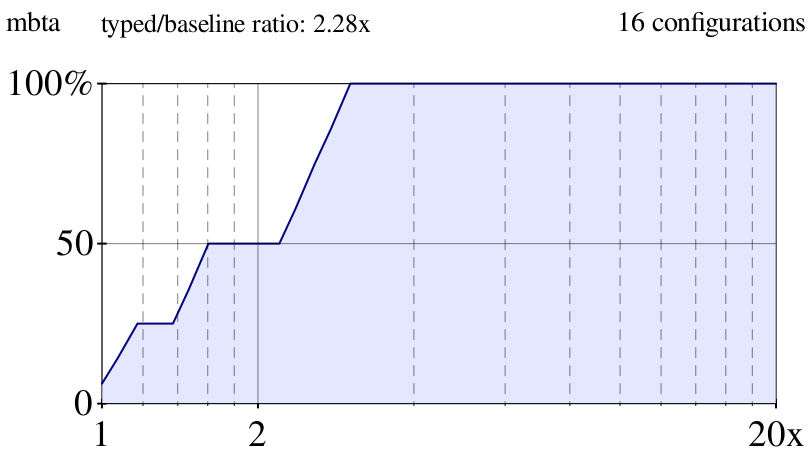(parameterize ((*OVERHEAD-SHOW-RATIO* #f)) (overhead-plot (list mbta (typed-racket-info%best-typed-path mbta 2))))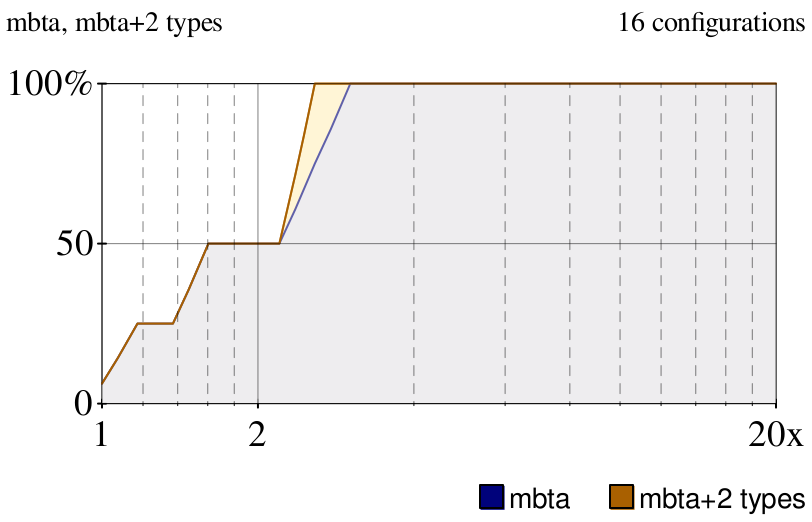procedure(exact-runtime-plot pi) → pict? pi : (treeof performance-info?)
Plots all runtimes for all configurations. The x-axis is the number of type annotations in the configuration, the y-axis is its running time.

One configuration cfg renders N points, where N is the length of the list (configuration-info->runtime* cfg). These points are spread across the x-axis bucket that represents configurations with T type annotations, where T is the value of (configuration-info->num-types cfg).

(exact-runtime-plot mbta)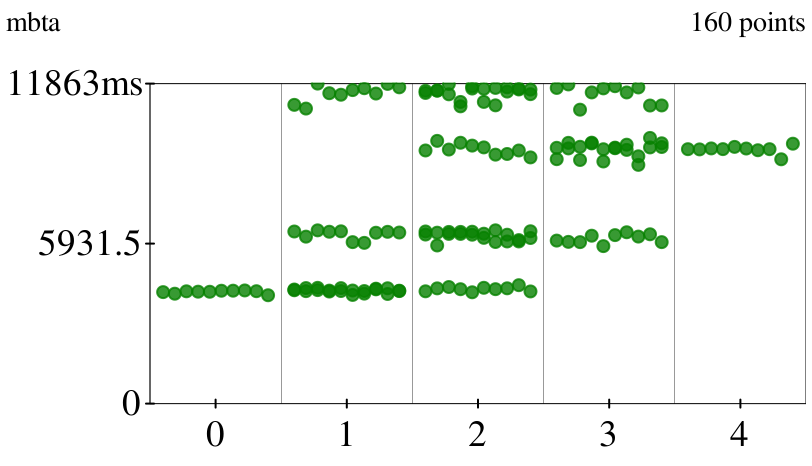procedure(samples-plot si) → pict? si : sample-info?
Similar to overhead-plot, but intended for SRA performance info structures. Plots a 95% confidence interval for the number of configurations that run with at most Dx overhead.

(parameterize ([*OVERHEAD-SHOW-RATIO* #f]) (samples-plot sample_fsm))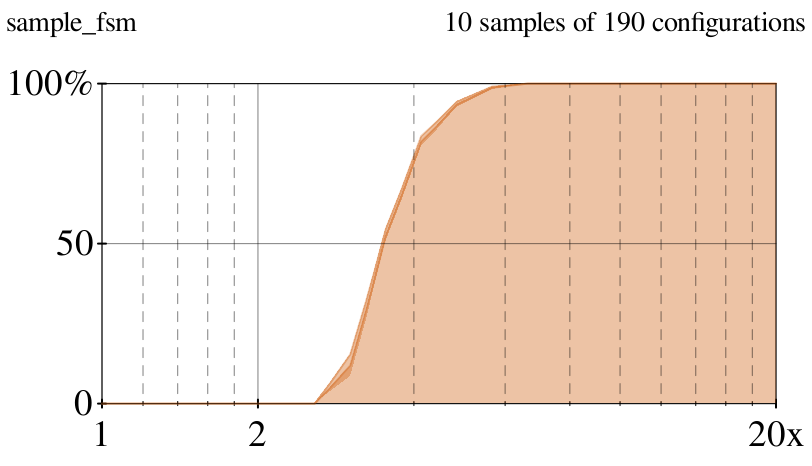procedure(validate-samples-plot pi si) → pict? pi : performance-info? si : sample-info?
Plot exhaustive and approximate data side-by-side.

 (parameterize ([*OVERHEAD-SHOW-RATIO* #f]) (let* ((sample* (for/list ((i (in-range 4))) (random-sample (in-configurations mbta) 20))) (si (make-sample-info mbta sample*))) (validate-samples-plot mbta si)))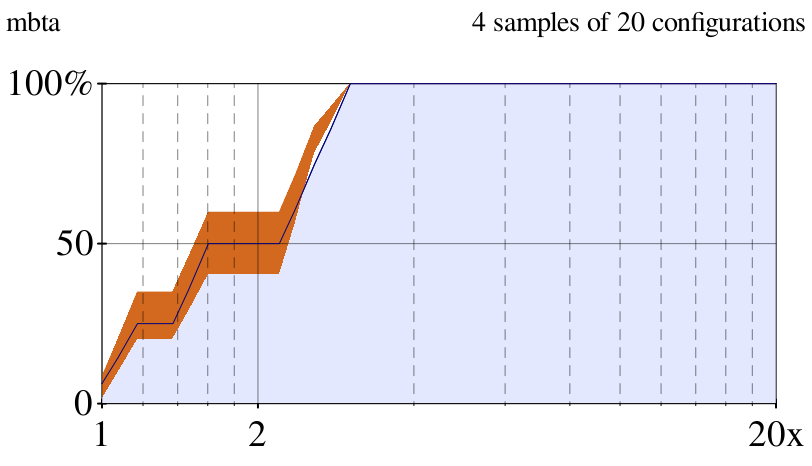procedure(relative-scatterplot pi-x pi-y) → pict? pi-x : performance-info? pi-y : performance-info?
Plots points (X, Y) where X is the overhead of a configuration in pi-x and Y is the overhead of the same configuration in pi-y. Assumes pi-x and pi-y are two datasets for the same benchmark.

Points along the line X=Y represent configurations with equal overhead in both datasets. Points below the line are faster in pi-x and points to the left of the line are faster in pi-y.

 (parameterize ([*POINT-SYMBOL* 'dot] [*POINT-SIZE* 30] [*POINT-ALPHA* 0.5] [*POINT-COLOR* 6]) (relative-scatterplot mbta mbta))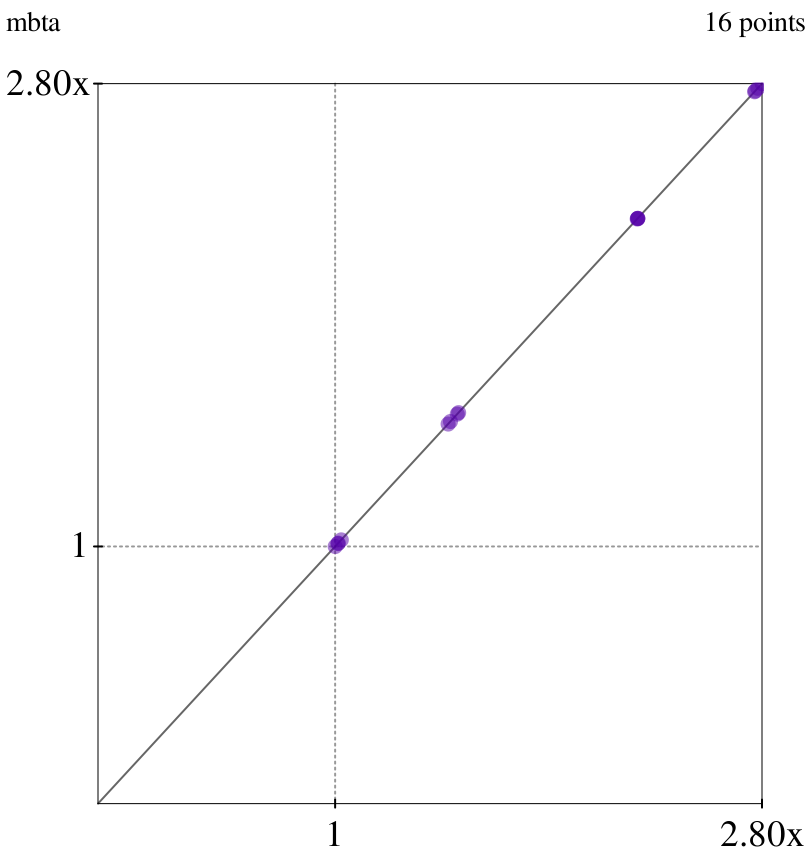Added in version 0.2 of package gtp-plot.

 procedure(grid-plot make-plot data*) → pict? make-plot : (-> any/c pict?) data* : (listof any/c)
Build plots for a sequence of datasets and arrange the plots into a grid.

 (parameterize ([*FONT-SIZE* 8] [*GRID-NUM-COLUMNS* 2] [*GRID-Y* #f] [*OVERHEAD-PLOT-HEIGHT* 100] [*OVERHEAD-SHOW-RATIO* #f]) (grid-plot overhead-plot (list mbta mbta mbta)))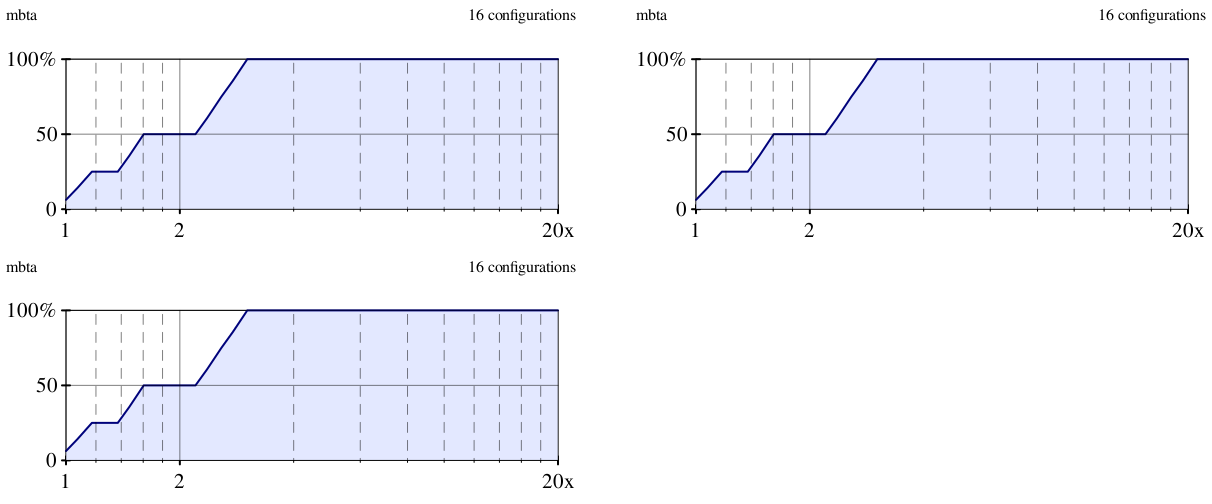procedure(performance-lattice pi) → pict? pi : (or/c performance-info? natural?)
Given a performance-info structure, shows the overhead of every configuration in a lattice. Given a number, render an unlabeled lattice.

 (parameterize ([*FONT-SIZE* 14] [*LATTICE-UNIT-WIDTH* 16] [*LATTICE-UNIT-HEIGHT* 12] [*LATTICE-CONFIG-X-MARGIN* 10] [*LATTICE-CONFIG-Y-MARGIN* 25] [*LATTICE-LINES?* #true] [*LATTICE-LINE-ALPHA* 0.5]) (ht-append 4 (performance-lattice mbta) (performance-lattice 3)))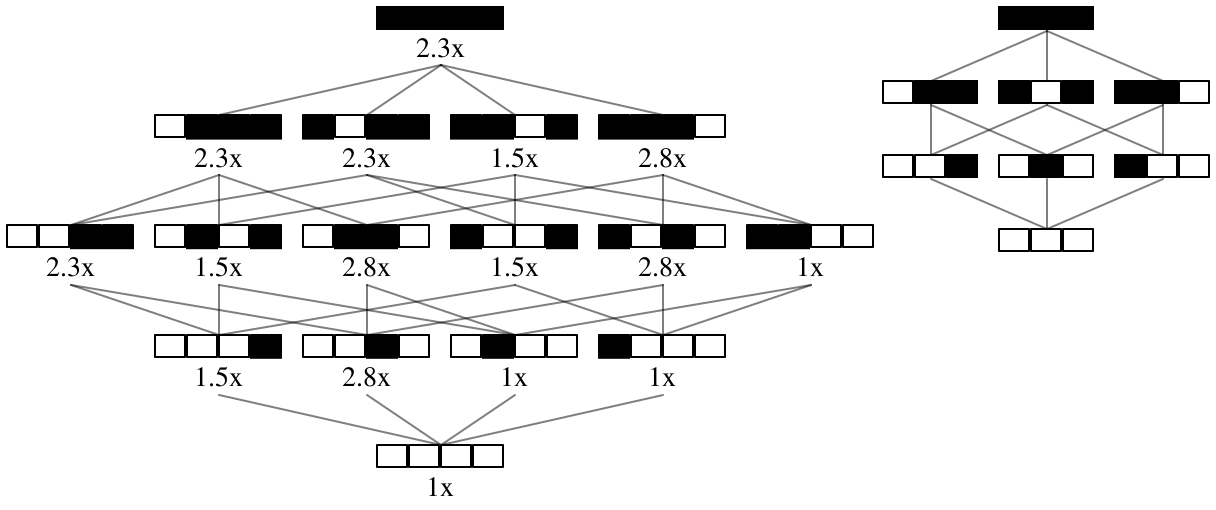Added in version 0.5 of package gtp-plot.

 procedure(configuration-lattice pi) → pict? pi : performance-info?
Show the overhead of every configuration in a lattice.

 (parameterize ([*FONT-SIZE* 14] [*LATTICE-UNIT-WIDTH* 16] [*LATTICE-UNIT-HEIGHT* 12] [*LATTICE-CONFIG-Y-MARGIN* 24] [*LATTICE-LINES?* #true] [*LATTICE-LINE-ALPHA* 0.5]) (performance-lattice mbta))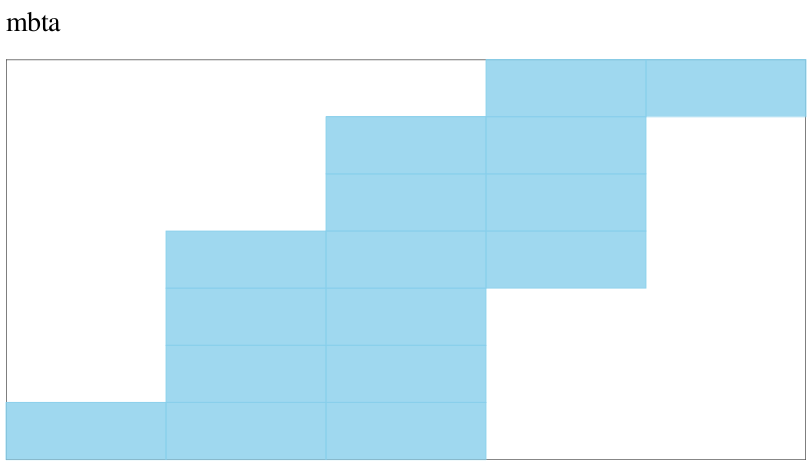Added in version 0.5 of package gtp-plot.

#### 3.1Experimental Plotting Functions

The functions in this section make strange plots. The appearance of these plots is subject to change.

 procedure(cloud-plot pi) → pict? pi : performance-info?
Plots a heatmap showing the order-of-magnitude of the overhead of each configuration.

Intended for exhaustive performance info structures.

(cloud-plot mbta)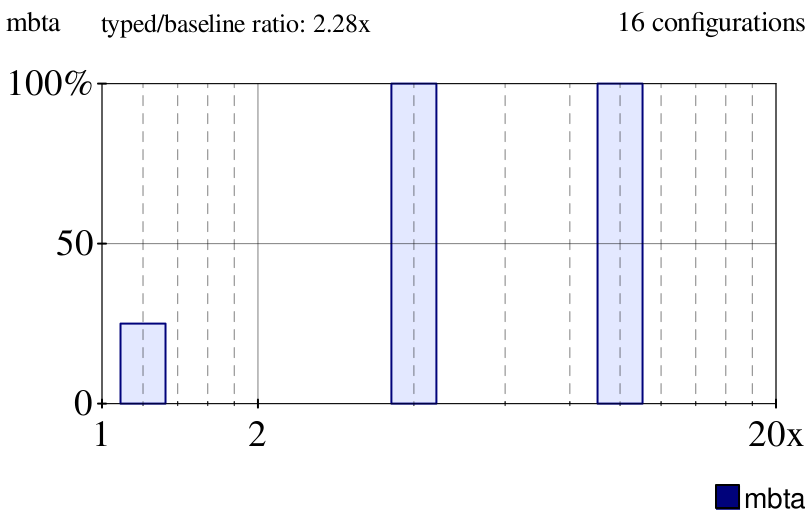procedure(discrete-overhead-plot pi D*) → pict? pi : performance-info? D* : (listof nonnegative-real/c)
Plots a histogram of the number of D-deliverable configurations for each D in the given list.

(discrete-overhead-plot mbta '(1.2 4 10))procedure(rectangle-plot pi) → pict? pi : performance-info?
Plots a thermometer showing the proportion of configurations that run within Dx overhead, where D is the value of (*STANDARD-D*).

Intended for exhaustive performance info structures.

(rectangle-plot mbta)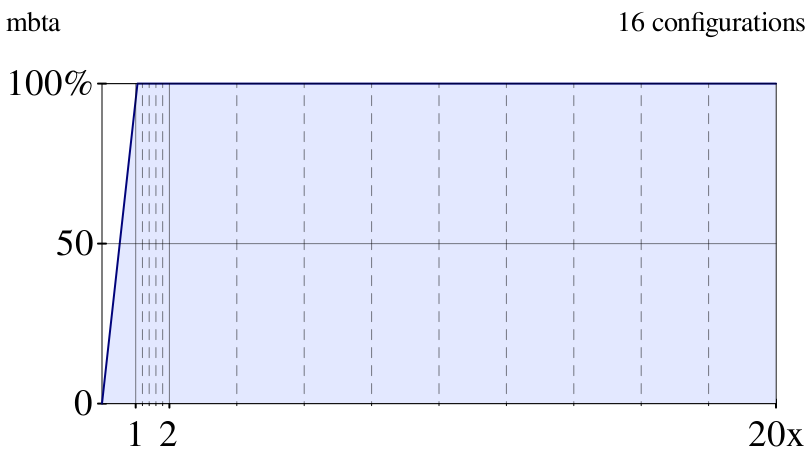procedure(relative-overhead-cdf pi-base pi-new) → pict? pi-base : performance-info? pi-new : performance-info?
Plot a CDF with the relative overhead of pi-new vs. pi-base. More precisely:
• finds the configurations that are common to pi-new and pi-base;

• groups the configurations by the quotient of their pi-new runtime and pi-base runtime;

• plots a line of points (x,y), which means: y% of the common configurations are at most x times slower on pi-new

If pi-old and pi-new are the same, then the relative overhead of each configuration is 1.

(relative-overhead-cdf mbta mbta)#### 3.2Plot Parameters

parameter

(*BAR-WIDTH*)  nonnegative-real/c

(*BAR-WIDTH* bar-width)  void?
bar-width : nonnegative-real/c
 = 0.1
Controls width of bars in a discrete-overhead-plot.

parameter

(*DECORATIONS-COLOR*)  plot-color/c

(*DECORATIONS-COLOR* pc)  void?
pc : plot-color/c
 = 0
Sets color of some plot decorations

Added in version 0.2 of package gtp-plot.

parameter

(*FONT-SIZE*)  exact-positive-integer?

(*FONT-SIZE* font-size)  void?
font-size : exact-positive-integer?
 = 10
Controls font size of text in plots.

parameter

(*GRID-X*)  (or/c #f natural?)

(*GRID-X* grid-x)  void?
grid-x : (or/c #f natural?)
 = 600
Controls the width of a grid-plot. If #false, the width of the grid is at least (* (*GRID-NUM-COLUMNS*) (*OVERHEAD-PLOT-WIDTH*)).

parameter

(*GRID-Y*)  (or/c #f natural?)

(*GRID-Y* grid-y)  void?
grid-y : (or/c #f natural?)
 = 1300
Controls the height of a grid-plot. If #false, the height of the grid is at least (* (quotient N (*GRID-NUM-COLUMNS*)) (*OVERHEAD-PLOT-WIDTH*)), where N is the number of plots in the grid.

parameter

(*GRID-X-SKIP*)  natural?

(*GRID-X-SKIP* grid-x-skip)  void?
grid-x-skip : natural?
 = 30
Horizontal space between plots on a grid-plot.

parameter

(*GRID-Y-SKIP*)  natural?

(*GRID-Y-SKIP* grid-y-skip)  void?
grid-y-skip : natural?
 = 6
Vertical space between plots on a grid-plot.

parameter

(*GRID-NUM-COLUMNS*)  exact-positive-integer?

(*GRID-NUM-COLUMNS* grid-num-columns)  void?
grid-num-columns : exact-positive-integer?
 = 3
Number of columns on a grid-plot.

parameter

(*INTERVAL-ALPHA*)  nonnegative-real/c

(*INTERVAL-ALPHA* ia)  void?
ia : nonnegative-real/c
 = 1
Sets the transparency of shaded plot regions.

parameter

(*LEGEND-Y-SKIP*)  real?

(*LEGEND-Y-SKIP* n)  void?
n : real?
 = 10
Vertical space between legend pict and a plot.

parameter

(*OVERHEAD-FREEZE-BODY*)  boolean?

(*OVERHEAD-FREEZE-BODY* freeze?)  void?
freeze? : boolean?
 = #f
When true, plotting functions will call freeze on rendered plots before returning. This is useful for plots with a large number of elements.

parameter

(*OVERHEAD-LEGEND?*)  boolean?

(*OVERHEAD-LEGEND?* show-legend?)  void?
show-legend? : boolean?
 = #true
When #false, hide dataset name and axis labels.

parameter

(*OVERHEAD-LINE-COLOR*)  plot-color/c

(*OVERHEAD-LINE-COLOR* line-color)  void?
line-color : plot-color/c
 = 3
Sets color of solid lines used in plots.

parameter

(*OVERHEAD-LINE-WIDTH*)  nonnegative-real/c

(*OVERHEAD-LINE-WIDTH* line-width)  void?
line-width : nonnegative-real/c
 = 1
Sets width of solid lines used in plots.

parameter

(*OVERHEAD-MAX*)  exact-positive-integer?

(*OVERHEAD-MAX* x-max)  void?
x-max : exact-positive-integer?
 = 20
Sets maximum x-value in overhead plots.

parameter

(*OVERHEAD-PLOT-HEIGHT*)  nonnegative-real/c

(*OVERHEAD-PLOT-HEIGHT* plot-height)  void?
plot-height : nonnegative-real/c
 = 300

parameter

(*OVERHEAD-PLOT-WIDTH*)  nonnegative-real/c

(*OVERHEAD-PLOT-WIDTH* plot-height)  void?
plot-height : nonnegative-real/c
 = 600
Sets height and width of plots.

parameter

(*OVERHEAD-SAMPLES*)  exact-positive-integer?

(*OVERHEAD-SAMPLES* num-samples)  void?
num-samples : exact-positive-integer?
 = 20
Number of points used to draw the solid color line in overhead plots.

parameter

(*OVERHEAD-SHOW-RATIO*)  boolean?

(*OVERHEAD-SHOW-RATIO* show?)  void?
show? : boolean?
 = #t
If true, plots come with a typed/baseline ratio.

parameter

(*POINT-ALPHA*)  nonnegative-real/c

(*POINT-ALPHA* pa)  void?
pa : nonnegative-real/c
 = 0.4
Sets translucency of points.

parameter

(*POINT-COLOR*)  plot-color/c

(*POINT-COLOR* pc)  void?
pc : plot-color/c
 = 2
Sets color of points.

parameter

(*POINT-SIZE*)  exact-positive-integer?

(*POINT-SIZE* ps)  void?
ps : exact-positive-integer?
 = 3
Sets size of points.

parameter

(*POINT-SYMBOL*)  point-sym/c

(*POINT-SYMBOL* ps)  void?
ps : point-sym/c
 = 'fullcircle
Symbol used to draw points.

parameter

(*SAMPLE-COLOR*)  plot-color/c

(*SAMPLE-COLOR* sc)  void?
sc : plot-color/c
 = "chocolate"
Color used to draw sample plots.

parameter

(*STANDARD-D*)  (or/c #f positive?)

(*STANDARD-D* D)  void?
D : (or/c #f positive?)
 = #f
A default overhead value.

parameter

(*LATTICE-UNIT-BORDER-WIDTH*)  nonnegative-real?

(*LATTICE-UNIT-BORDER-WIDTH* r)  void?
r : nonnegative-real?
 = 0
Border width for each little square (unit) in a lattice.

parameter

(*LATTICE-UNIT-X-MARGIN*)  nonnegative-real?

(*LATTICE-UNIT-X-MARGIN* r)  void?
r : nonnegative-real?
 = 0
Space between units in one configuration rectangle.

parameter

(*LATTICE-UNIT-HEIGHT*)  nonnegative-real?

(*LATTICE-UNIT-HEIGHT* r)  void?
r : nonnegative-real?
 = 6
Height of units in a configuration. This is also the height of the configuration.

parameter

(*LATTICE-UNIT-WIDTH*)  nonnegative-real?

(*LATTICE-UNIT-WIDTH* r)  void?
r : nonnegative-real?
 = 3
Width of units in a configuration.

parameter

(*LATTICE-CONFIG-X-MARGIN*)  nonnegative-real?

(*LATTICE-CONFIG-X-MARGIN* r)  void?
r : nonnegative-real?
 = 3
Horizontal space between configurations on the same level of a lattice.

parameter

(*LATTICE-CONFIG-Y-MARGIN*)  nonnegative-real?

(*LATTICE-CONFIG-Y-MARGIN* r)  void?
r : nonnegative-real?
 = 8
Vertical space between levels of a lattice.

parameter

(*LATTICE-CONFIG-LABEL-MARGIN*)  nonnegative-real?

(*LATTICE-CONFIG-LABEL-MARGIN* r)  void?
r : nonnegative-real?
 = 1
Space between a configuration and the label right below.

parameter

(*LATTICE-LINES?*)  boolean?

(*LATTICE-LINES?* r)  void?
r : boolean?
 = #false
When true, draw lines between configurations that are one type-migration step apart.

parameter

(*LATTICE-LINE-WIDTH*)  nonnegative-real?

(*LATTICE-LINE-WIDTH* r)  void?
r : nonnegative-real?
 = 0.5
Width of lattice lines. Irrelevant if (*LATTICE-LINES?*) is false.

parameter

(*LATTICE-LINE-ALPHA*)  nonnegative-real?

(*LATTICE-LINE-ALPHA* r)  void?
r : nonnegative-real?
 = 0.2
Opacity of lattice lines.

parameter

(*LATTICE-UNTYPED-COLOR*)  plot-color/c

(*LATTICE-UNTYPED-COLOR* c)  void?
c : plot-color/c
 = "white"
Color for untyped units in a lattice.

parameter

(*LATTICE-TYPED-COLOR*)  plot-color/c

(*LATTICE-TYPED-COLOR* c)  void?
c : plot-color/c
 = "black"
Color for typed units in a lattice.

### 4Data Formats

#### 4.1Typed Racket Info

 (require gtp-plot/typed-racket-info) package: gtp-plot

 procedure ps : path-string?
A Typed Racket dataset:
• lives in a file named "NAME-vX-OTHER.rktd", where "NAME" is the name of the program and "vX" is a Racket version number, and "OTHER" is any string (used to distinguish this data from other data with the same prefix).

or lives in a file with any name where the first non-whitespace characters on the first non-blank-line, non-comment line are the #( characters.

or lives in a #lang gtp-measure/output/typed-untyped file.

• contains (or is) a Racket vector with (expt 2 N) elements (for some natural N); entries in the vector are lists of runtimes.

or contains valid #lang gtp-measure/output/typed-untyped data.

Changed in version 0.4 of package gtp-plot: Accept gtp-measure output.
Changed in version 0.5: Accept vector

Example data:
 ;; Example Typed Racket GTP dataset #((1328) (42) (8) (1))

 procedure(make-typed-racket-info ps [#:name name]) → typed-racket-info? ps : typed-racket-data? name : (or/c #f symbol?) = #f
Build a performance info structure from a Typed Racket dataset.

 procedure x : any/c
Predicate for Typed Racket datasets.

 procedure x : any/c
Predicate for a Typed Racket configuration name. A name is a k-character string of #\0 and #\1 characters. Each digit represents one unit, which may or may not be typed. A #\1 means typed and a #\0 means untyped.

Examples:
 > (typed-racket-id? "0000") '(#\0 #\1) > (typed-racket-id? "001000011") '(#\1) > (typed-racket-id? "2001") #f > (typed-racket-id? 10) #f

 procedure(typed-racket-id<=? id0 id1) → boolean? id0 : typed-racket-id? id1 : typed-racket-id?
Returns true if the first id describes a subset of the types in the second id.

Examples:
 > (typed-racket-id<=? "0000" "1111") #t > (typed-racket-id<=? "1111" "0000") #f > (typed-racket-id<=? "0110" "1110") #t > (typed-racket-id<=? "0110" "1011") #f

Added in version 0.6 of package gtp-plot.

procedure

 (make-typed-racket-sample-info src #:name name #:typed-configuration tc #:untyped-configuration uc)
sample-info?
src : (listof typed-racket-info?)
name : symbol?
tc : typed-configuration-info?
uc : untyped-configuration-info?
Make a sample-info? structure for a Typed Racket program, given a list of data files for the program (src), a name for the program name, and data for the program’s typed and untyped configurations.

procedure

 (make-typed-racket-configuration-info id time*)
typed-racket-configuration-info?
id : typed-racket-id?
time* : (listof nonnegative-real/c)
Constructor for a Typed Racket configuration.

 procedure x : any/c
Predicate for a Typed Racket configuration.

 procedure x : any/c
Predicate for a fully-typed Typed Racket configuration.

 procedure x : any/c
Predicate for an untyped Typed Racket configuration.

 procedure pi : typed-racket-info? n : natural?
Return a structure with the same configurations as pi, but each configuration has the best-possible runtimes that can be reached after n type conversion steps.

Added in version 0.6 of package gtp-plot.

#### 4.2Reticulated Info

 (require gtp-plot/reticulated-info) package: gtp-plot

 procedure ps : path-string?
A Reticulated dataset is a path to a directory. The directory:
• has a name like "NAME-OTHER", where "NAME" is the name of the program and "OTHER" is any string (used to distinguish from other data with the same prefix);

• contains a file named "NAME-python.tab", where each line has a floating point number;

• either:

A Reticulated data file describes the running time of configurations. Each line in a data file has the format:
 ID NUM-TYPES [TIME, ...]
where:
• ID is a hyphen-separated sequence of digits. These represent a configuration as a mixed-radix number; the radix of position k is the number of typeable components in module k of the program.

• NUM-TYPES is a natural number, and

• TIME is a positive floating-point number.

Two lines from an example Reticulated data file:
 13-0-2-7-7 9 [2.096725507, 2.134529453, 2.088266101, 2.1067140589999998, ] 0-1-18-7-3 11 [2.153331553, 2.217980131, 2.0789024329999997, 2.1293707979999996, ]

A Reticulated meta file states the number of type annotations in a sampled program via a Racket hash. Example meta file:
 #hash((num-units . 19))

Sorry for the complex format, it’s strongly motivated by the data in the gm-pepm-2018 package.

 procedure(make-reticulated-info ps [kind]) → reticulated-info? ps : reticulated-data? kind : (or/c 'exhaustive 'approximate #f) = #f
Build a performance info structure from a Reticulated dataset.

By default, inspects the given dataset and chooses whether to return a reticulated-info? struct with exhaustive data or a sample-info? struct with approximate data. Use the kind argument to override the default.

 procedure x : any/c
Predicate for Reticulated datasets.

 procedure x : any/c
Predicate for a Reticulated configuration name. A name is a sequence of base-10 numbers. Each position in the sequence corresponds to a module. Each number encodes the type annotations in the module.

Configuration names may be strings of hypen-separated numbers or lists of natural numbers.

Examples:
 > (reticulated-id? "0") #t > (reticulated-id? "0-0-0") #t > (reticulated-id? "2001-14-3") #t > (reticulated-id? '(2001 14 3)) #t

To decode the type annotations from one number N, convert N to base-2 and look for #\0 digits. Each place in a binary number correponds to a typed unit, each #\0 means the unit is typed, and each #\1 means the unit in untyped.

Be advised, the interpretations of #\0 and #\1 are the opposite of what typed-racket-id? does.

 procedure(reticulated-id<=? id0 id1) → boolean? id0 : reticulated-id? id1 : reticulated-id?
Returns true if the first configuration name describes a subset of the type annotations in the second name.

Examples:
 > (reticulated-id<=? "1" "0") #t > (reticulated-id<=? "0" "1") #f > (reticulated-id<=? "7-6" "5-6") #t > (reticulated-id<=? "6-6" "5-6") #f

The input configurations must be for the same benchmark.

Added in version 0.6 of package gtp-plot.

 procedure pi : reticulated-info? n : natural?
Return a structure with the same configurations as pi, but each configuration has the best-possible runtimes that can be reached after n type conversion steps.

Added in version 0.6 of package gtp-plot.

### 5Support

 (require gtp-plot) package: gtp-plot

#### 5.1System API

 (require gtp-plot/system) package: gtp-plot
Convenience API for making system calls.

NOTE: This library is deprecated; use gtp-util, instead.

See also racket/system.

 procedure(shell cmd arg* ...) → string? cmd : path-string? arg* : (or/c path-string? (listof path-string?))
Finds the executable that cmd denotes, then invokes it with the given arguments. Returns a string containing all output from the system call. Raises an exn:fail:user? exception if the executable exits uncleanly.

 procedure(md5sum filename) → string? filename : path-string?
Same as (call-with-input-file filename md5).

#### 5.2Other Helper Functions

 (require gtp-plot/util) package: gtp-plot

 syntax
 syntax
 syntax
 syntax
 syntax
 syntax
Subscribe to gtp-logger for diagnostics.

See also: define-logger.

NOTE: This sequence of bindings from here down is deprecated; use gtp-util, instead. Keep using gtp-plot/util for the logger above.

 procedure x : any/c
Flat contract for non-negative real numbers.

procedure

 (confidence-interval r* [ #:cv confidence-value])
(cons/c real? real?)
r* : (listof real?)
confidence-value : nonnegative-real/c = 1.96
Return a 95% confidence interval for the given numbers at the given confidence value.

 procedure(tab-split str) → (listof string?) str : string?
Split a string by tab characters.

 procedure(tab-join str*) → string? str* : (listof string?)
Join a list of strings by tab characters.

 procedure ps : path-string?
Convert a path or string to a string.

 procedure ps : path-string?
If the given directory exists, do nothing. Otherwise, create it.

 procedure(rnd r) → string? r : real?
Round the given number to two decimal places.

 procedure(pct a b) → real? a : real? b : real?
Same as (* 100 (/ a b)).

 procedure(log2 n) → natural? n : natural?
Compute the base-2 logarithm of a number.

Assumes n is a power of 2.

 procedure n : real?
Count the number of digits in the given number.

 procedure ps : path-string?
Remove the extension from the given filename.

 procedure(save-pict out-path p) → boolean? out-path : path-string? p : pict?
Write the given pict to the given filename in .png format.

 procedure(columnize x* num-cols) → (listof list?) x* : list? num-cols : natural?
Divide a list into almost-equally-sized lists.

procedure

(force/cpu-time thunk)
 any/c natural?
thunk : (-> any)
Force the given thunk and record its running time. Return both the result of the thunk and the CPU time (as reported by time-apply).

 procedure(natural->bitstring n #:pad pad) → string? n : natural? pad : natural?
Return a binary representation of n with pad bits.

 procedure str : string?
Parse a string of "1" and "0" digits as a binary number, return the base-10 representation of the parsed number.

 procedure(count-zero-bits str) → natural? str : string?
Count the number of #\0 characters in a string of "1" and "0" digits.

### 6GTP Glossary

(These aren’t definitions, these are examples.)

A typeable component in Typed Racket is a module. A typeable component in Reticulated is a function parameter, function return, or class field (see def and @fields).

A configuration is a gradually-typed program. Given a fully-typed program with N typeable components, there are (expt 2 N) configurations that have fewer annotations than the fully-typed configuration.